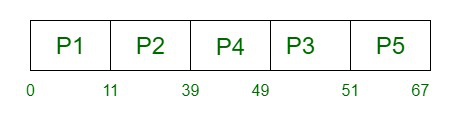# Priority CPU Scheduling with different arrival time – Set 2

• Difficulty Level : Medium
• Last Updated : 08 Dec, 2021

Prerequisite – Program for Priority Scheduling – Set 1
Priority scheduling is a non-preemptive algorithm and one of the most common scheduling algorithms in batch systems. Each process is assigned first arrival time (less arrival time process first) if two processes have same arrival time, then compare to priorities (highest process first). Also, if two processes have same priority then compare to process number (less process number first). This process is repeated while all process get executed.

Implementation –

1. First input the processes with their arrival time, burst time and priority.
2. First process will schedule, which have the lowest arrival time, if two or more processes will have lowest arrival time, then whoever has higher priority will schedule first.
3. Now further processes will be schedule according to the arrival time and priority of the process. (Here we are assuming that lower the priority number having higher priority). If two process priority are same then sort according to process number.
Note: In the question, They will clearly mention, which number will have higher priority and which number will have lower priority.
4. Once all the processes have been arrived, we can schedule them based on their priority.Gantt Chart –Examples –

```Input :
process no-> 1 2 3 4 5
arrival time-> 0 1 3 2 4
burst time-> 3 6 1 2 4
priority-> 3 4 9 7 8
Output :
Process_no   arrival_time   Burst_time   Complete_time    Turn_Around_Time       Waiting_Time
1             0               3                3                   3               0
2             1               6                9                   8               2
3             3               1                16                  13              12
4             2               2                11                  9               7
5             4               4                15                  11              7
Average Waiting Time is : 5.6
Average Turn Around time is : 8.8 ```

## C++

 `// C++ implementation for Priority Scheduling with``//Different Arrival Time priority scheduling``/*1. sort the processes according to arrival time``2. if arrival time is same the acc to priority``3. apply fcfs``*/` `#include ` `using` `namespace` `std;` `#define totalprocess 5` `// Making a struct to hold the given input` `struct` `process``{``int` `at,bt,pr,pno;``};` `process proc;` `/*``Writing comparator function to sort according to priority if``arrival time is same``*/` `bool` `comp(process a,process b)``{``if``(a.at == b.at)``{``return` `a.pr

## Java

 `// Java implementation for Priority Scheduling with``//Different Arrival Time priority scheduling``import` `java.util.*;` `/// Data Structure``class` `Process {``    ``int` `at, bt, pri, pno;``    ``Process(``int` `pno, ``int` `at, ``int` `bt, ``int` `pri)``    ``{``        ``this``.pno = pno;``        ``this``.pri = pri;``        ``this``.at = at;``        ``this``.bt = bt;``    ``}``}` `/// Gantt chart structure``class` `GChart {``    ``// process number, start time, complete time,``    ``// turn around time, waiting time``    ``int` `pno, stime, ctime, wtime, ttime;``}` `// user define comparative method (first arrival first serve,``// if arrival time same then heigh priority first)``class` `MyComparator ``implements` `Comparator {` `    ``public` `int` `compare(Object o1, Object o2)``    ``{` `        ``Process p1 = (Process)o1;``        ``Process p2 = (Process)o2;``        ``if` `(p1.at < p2.at)``            ``return` `(-``1``);` `        ``else` `if` `(p1.at == p2.at && p1.pri > p2.pri)``            ``return` `(-``1``);` `        ``else``            ``return` `(``1``);``    ``}``}`  `// class to find Gantt chart``class` `FindGantChart {``    ``void` `findGc(LinkedList queue)``    ``{` `        ``// initial time = 0``        ``int` `time = ``0``;` `        ``// priority Queue sort data according``        ``// to arrival time or priority (ready queue)``        ``TreeSet prique = ``new` `TreeSet(``new` `MyComparator());` `        ``// link list for store processes data``        ``LinkedList result = ``new` `LinkedList();` `        ``// process in ready queue from new state queue``        ``while` `(queue.size() > ``0``)``            ``prique.add((Process)queue.removeFirst());` `        ``Iterator it = prique.iterator();` `        ``// time set to according to first process``        ``time = ((Process)prique.first()).at;` `        ``// scheduling process``        ``while` `(it.hasNext()) {` `            ``// dispatcher dispatch the``            ``// process ready to running state``            ``Process obj = (Process)it.next();` `            ``GChart gc1 = ``new` `GChart();``            ``gc1.pno = obj.pno;``            ``gc1.stime = time;``            ``time += obj.bt;``            ``gc1.ctime = time;``            ``gc1.ttime = gc1.ctime - obj.at;``            ``gc1.wtime = gc1.ttime - obj.bt;` `            ``/// store the exxtreted process``            ``result.add(gc1);``        ``}` `        ``// create object of output class and call method``        ``new` `ResultOutput(result);``    ``}``}`

## Python3

 `# Python3 implementation for Priority Scheduling with``# Different Arrival Time priority scheduling``"""1. sort the processes according to arrival time``   ``2. if arrival time is same the acc to priority``   ``3. apply fcfs """`` ` `totalprocess ``=` `5``proc ``=` `[]``for` `i ``in` `range``(``5``):``    ``l ``=` `[]``    ``for` `j ``in` `range``(``4``):``        ``l.append(``0``)``    ``proc.append(l)` `# Using FCFS Algorithm to find Waiting time``def` `get_wt_time( wt):` `    ``# declaring service array that stores``    ``# cumulative burst time``    ``service ``=` `[``0``] ``*` `5` `    ``# Initialising initial elements``    ``# of the arrays``    ``service[``0``] ``=` `0``    ``wt[``0``] ``=` `0` `    ``for` `i ``in` `range``(``1``, totalprocess):``        ``service[i] ``=` `proc[i ``-` `1``][``1``] ``+` `service[i ``-` `1``]``        ``wt[i] ``=` `service[i] ``-` `proc[i][``0``] ``+` `1` `        ``# If waiting time is negative,``        ``# change it o zero``        ``if``(wt[i] < ``0``) :    ``            ``wt[i] ``=` `0``        ` `def` `get_tat_time(tat, wt):` `    ``# Filling turnaroundtime array``    ``for` `i ``in` `range``(totalprocess):``        ``tat[i] ``=` `proc[i][``1``] ``+` `wt[i]` `def` `findgc():``    ` `    ``# Declare waiting time and``    ``# turnaround time array``    ``wt ``=` `[``0``] ``*` `5``    ``tat ``=` `[``0``] ``*` `5` `    ``wavg ``=` `0``    ``tavg ``=` `0` `    ``# Function call to find waiting time array``    ``get_wt_time(wt)``    ` `    ``# Function call to find turnaround time``    ``get_tat_time(tat, wt)` `    ``stime ``=` `[``0``] ``*` `5``    ``ctime ``=` `[``0``] ``*` `5``    ``stime[``0``] ``=` `1``    ``ctime[``0``] ``=` `stime[``0``] ``+` `tat[``0``]``    ` `    ``# calculating starting and ending time``    ``for` `i ``in` `range``(``1``, totalprocess):``        ``stime[i] ``=` `ctime[i ``-` `1``]``        ``ctime[i] ``=` `stime[i] ``+` `tat[i] ``-` `wt[i]` `    ``print``(``"Process_no\tStart_time\tComplete_time"``,``               ``"\tTurn_Around_Time\tWaiting_Time"``)` `    ``# display the process details``    ``for` `i ``in` `range``(totalprocess):``        ``wavg ``+``=` `wt[i]``        ``tavg ``+``=` `tat[i]``        ` `        ``print``(proc[i][``3``], ``"\t\t"``, stime[i],``                         ``"\t\t"``, end ``=` `" "``)``        ``print``(ctime[i], ``"\t\t"``, tat[i], ``"\t\t\t"``, wt[i])`  `    ``# display the average waiting time``    ``# and average turn around time``    ``print``(``"Average waiting time is : "``, end ``=` `" "``)``    ``print``(wavg ``/` `totalprocess)``    ``print``(``"average turnaround time : "` `, end ``=` `" "``)``    ``print``(tavg ``/` `totalprocess)` `# Driver code``if` `__name__ ``=``=``"__main__"``:``    ``arrivaltime ``=` `[``1``, ``2``, ``3``, ``4``, ``5``]``    ``bursttime ``=` `[``3``, ``5``, ``1``, ``7``, ``4``]``    ``priority ``=` `[``3``, ``4``, ``1``, ``7``, ``8``]``    ` `    ``for` `i ``in` `range``(totalprocess):` `        ``proc[i][``0``] ``=` `arrivaltime[i]``        ``proc[i][``1``] ``=` `bursttime[i]``        ``proc[i][``2``] ``=` `priority[i]``        ``proc[i][``3``] ``=` `i ``+` `1``    ` `    ``# Using inbuilt sort function``    ``proc ``=` `sorted` `(proc, key ``=` `lambda` `x:x[``2``])``    ``proc ``=` `sorted` `(proc)``    ` `    ``# Calling function findgc for``    ``# finding Gantt Chart``    ``findgc()` `# This code is contributed by``# Shubham Singh(SHUBHAMSINGH10)`

Output:

```Process_no Start_time Complete_time Turn_Around_Time Waiting_Time
1           1           4              3              0
2           5           10             8              3
3           4           5              2              1
4          10           17             13             6
5          17           21             16             12
Average Waiting Time is : 4.4
Average Turn Around time is : 8.4```

Time Complexity: O(N * logN), where N is the total number of processes.
Auxiliary Space: O(N)

This article is contributed by Amit Verma . If you like GeeksforGeeks and would like to contribute, you can also write an article using contribute.geeksforgeeks.org or mail your article to contribute@geeksforgeeks.org. See your article appearing on the GeeksforGeeks main page and help other Geeks.